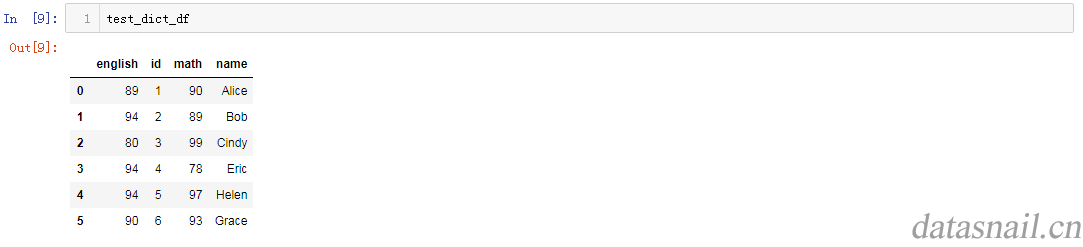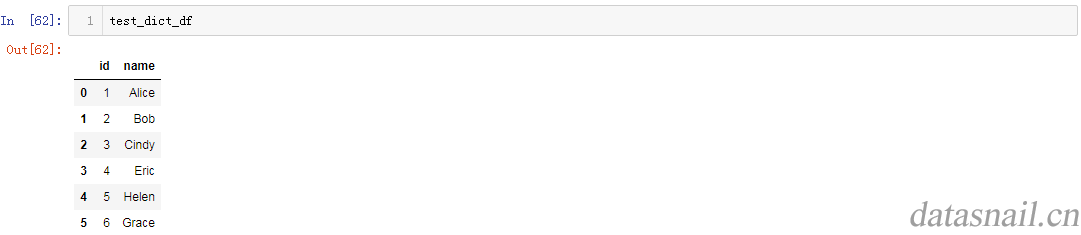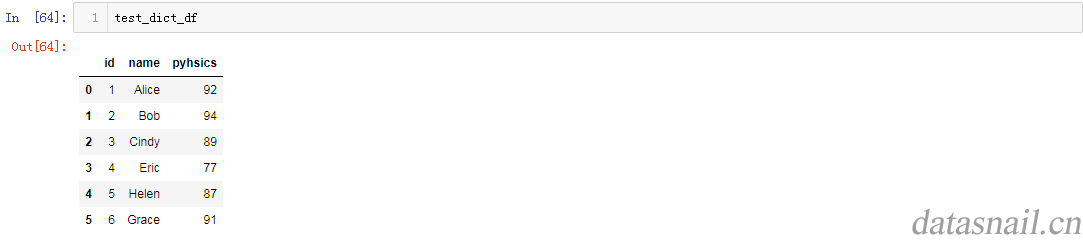# pandas DataFrame的创建方法

pandas DataFrame的增删查改总结系列文章：

①、把其他格式的数据整理到DataFrame中；
②在已有的DataFrame中插入N列或者N行。

#### 1. 字典类型读取到DataFrame（dict to DataFrame）

test_dict = {'id':[1,2,3,4,5,6],'name':['Alice','Bob','Cindy','Eric','Helen','Grace '],'math':[90,89,99,78,97,93],'english':[89,94,80,94,94,90]}
#.直接写入参数test_dict
test_dict_df = pd.DataFrame(test_dict)
#.字典型赋值
test_dict_df = pd.DataFrame(data=test_dict)test_dict_df = pd.DataFrame.from_dict(test_dict)


test_dict_df = pd.DataFrame({'id':1,'name':'Alice'}) # wrong style


test_dict_df = pd.DataFrame({'id':1,'name':'Alice'},pd.Index(range(1)))


pd.DataFrame({'id':[1,2],'name':['Alice','Bob']},pd.Index(range(2)))  #must be 2 in range function.


test_dict_df = pd.DataFrame(data=test_dict,columns=['id','name']) #only choose 'id' and 'name' columns


#### 2. csv文件构建DataFrame（csv to DataFrame）

df = pd.read_csv('./xxx.csv')


#### 3. 在已有的DataFrame中，增加N列或者N行3.1 添加列

new_columns = [92,94,89,77,87,91]
test_dict_df.insert(2,'pyhsics',new_columns)
#test_dict_df.insert(2,'pyhsics',new_columns,allow_duplicates=True)3.2 添加行

new_line = [7,'Iric',99]
test_dict_df.loc= new_line


test_dict_df.append(pd.DataFrame([new_line],columns=['id','name','physics']))


posted @ 2018-09-19 16:49  蒙面的普罗米修斯  阅读(82644)  评论(0编辑  收藏